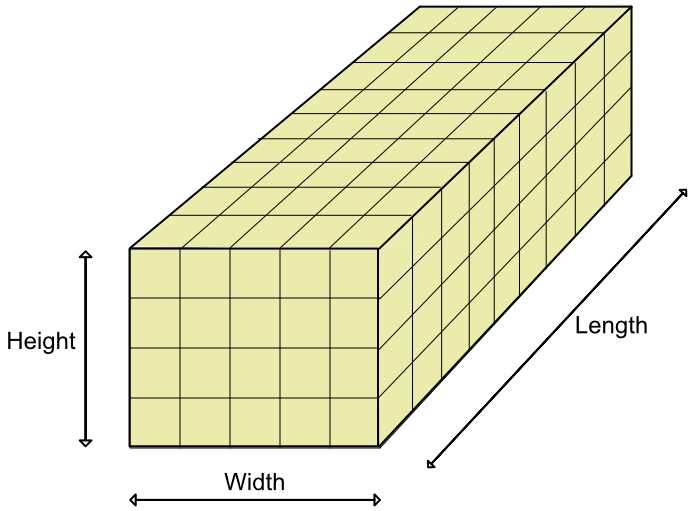Volume of a Cuboid

## Volume of a Cuboid

The volume of a cuboid is:

Volume = length x width x height

and is measured in cubic units e.g. mm3, cm3 or m3.

The cuboid shown below has been built from 1cm blocks. There are 9 x 5 x 4 (=180) blocks in the cuboid.## Example 1

What is the volume of a cuboid that is 20 cm by 30 cm, and is 1.2m long? Give the answer in m3.

Change the 20cm and 30cm measurements to metres

Volume of a cuboid = length x width x height

V = 0.2 x 0.3 x 1.2 = 0.072 m3

## Example 2

A building is 80m long, 20m wide and 10m high. The air conditioning units for the building can refresh the building at the rate of 5,000m3 per hour. The building manager wants all of the air in the building to be refreshed each hour.

How many air conditioning units should he buy?

The volume of a cuboid is length x width x height

V = 80 x 20 x 10 = 16000m3

The units refresh the air at 5000m3 per hour

16000 ÷ 5000 = 3.2; the manager will need 4 units.